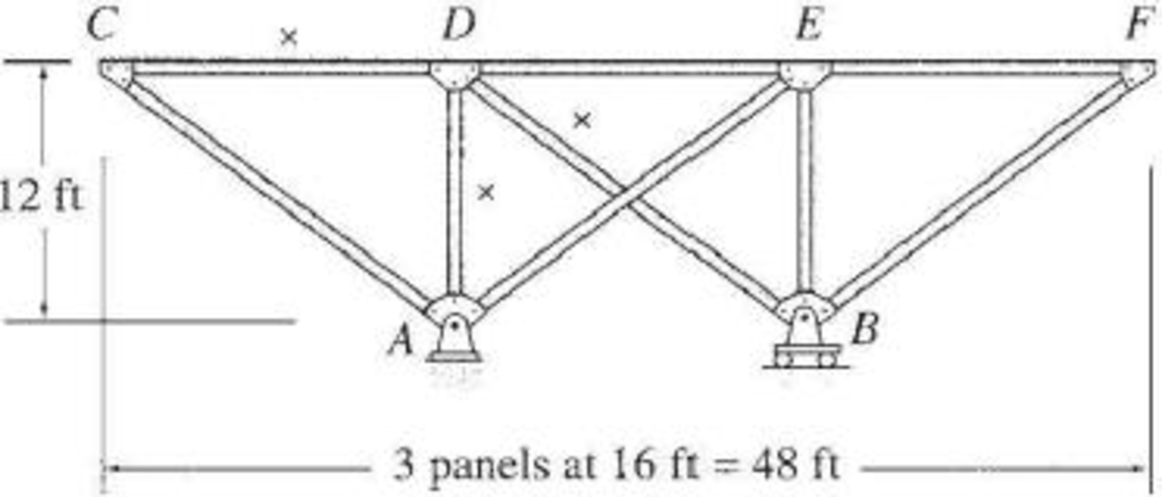# 8.53 through 8.57 Draw the influence lines for the forces in the members identified by an “×” of the trusses shown in Figs. P8.53–P8.57. Live loads are transmitted to the top chords of the trusses. FIG. P8.57

#### Solutions

Chapter
Section
Chapter 8, Problem 57P
Textbook Problem
5 views

## 8.53 through 8.57 Draw the influence lines for the forces in the members identified by an “×” of the trusses shown in Figs. P8.53–P8.57. Live loads are transmitted to the top chords of the trusses.FIG. P8.57To determine

Draw the influence lines for the force in member AD, BD, and CD.

### Explanation of Solution

Calculation:

Find the support reactions.

Apply 1 k moving load from C to F in the top chord member.

Draw the free body diagram of the member as in Figure 1.

Find the reaction at A and B when 1 k load placed from C to F. (0x48ft).

Apply moment equilibrium at A.

Consider clockwise moment as positive and anticlockwise moment as negative.

ΣMA=01(16x)=By(16)16By=x16By=x161

Apply vertical equilibrium.

ΣFy=0Ay+By=1Ay+x161=1Ay=2x16

Influence line for the force in member AB.

The expressions for the member force FBC can be determined by passing an imaginary section aa through member FG, BG, and BC and then apply a moment equilibrium at G.

Draw the free body diagram of the section aa as shown in Figure 3.

Refer Figure 2.

Slope of line AE is 4 horizontal and 3 vertical.

Find the equation of member force AD.

Apply a 1 k load at C (x=0).

Consider the section CD

Apply moment equilibrium equation at E.

Consider clockwise moment as positive and anticlockwise moment as negative.

Apply a 1 k load at just right of C (0ftx48ft).

Consider the section left of line aa.

Apply moment equilibrium equation at E.

Consider clockwise moment as positive and anticlockwise moment as negative.

Substitute 2x16 for Ay.

Thus, the equation of force in the member AD,

Find the force in member AD using the Equation (1) and (2) and then summarize the value in Table 1.

 x (ft) Apply 1 k load Force in member AD (k) Influence line ordinate for the force in member AD (k/k) 0 C 0 0 16 D 1616−2=−1 ‑1 32 E 0 0 48 F 1 1

Sketch the influence line diagram for ordinate for the force in member AD using Table 1 as shown in Figure 3.

Influence line for the force in member CD.

Refer Figure 4.

Find the force in member CD.

Apply 1 k load just the left of D (0x16ft)

Consider the section DF.

Apply moment equilibrium equation at A.

Consider clockwise moment as positive and anticlockwise moment as negative.

ΣMA=0FCD(12)By(16)=012FCD=16ByFCD=43By

Substitute x161 for By

### Still sussing out bartleby?

Check out a sample textbook solution.

See a sample solution

#### The Solution to Your Study Problems

Bartleby provides explanations to thousands of textbook problems written by our experts, many with advanced degrees!

Get Started

Find more solutions based on key concepts
Compute the nominal flexural strength of the composite beam in Problem 9.1-3. Use Fy=50 ksi.

Steel Design (Activate Learning with these NEW titles from Engineering!)

Present Example 9.4 in Chapter 9 using the format discussed in Section 4.2. Divide the example problem into Giv...

Engineering Fundamentals: An Introduction to Engineering (MindTap Course List)

What element is in stainless steel that makes it corrosion resistant?

Precision Machining Technology (MindTap Course List)

Describe how a chip can be used to locate a lost animal.

Enhanced Discovering Computers 2017 (Shelly Cashman Series) (MindTap Course List)

What formula can a project manager use to estimate task duration? Provide an example.

Systems Analysis and Design (Shelly Cashman Series) (MindTap Course List)

What types of tip seals are used with cutting torch tips?

Welding: Principles and Applications (MindTap Course List)

If your motherboard supports ECC DDR3 memory, can you substitute non-ECC DDR3 memory?

A+ Guide to Hardware (Standalone Book) (MindTap Course List)